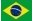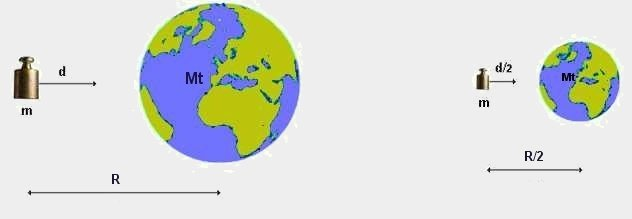Appendix 1 :Another way to understand the dimension of mass:If the distance decreases by half: A mass M situated at a distance R from the Earth undergoes a force of attraction F where F = K.m.Mt / R² Its acceleration towards the Earth is a = K.Mt / R² (acceleration = force divided by mass = F / m) Initially stationary, at the end of time T this mass m will have covered a distance of d = a.T²/2 = (K.Mt / R²).(T²/2) d = (K Mt / R²) (T²/2) or d R²= (K Mt ) (T²/2)         (1) If we decrease the distances (R and d) by half (as shown in the figure on the right), as if space was shrinking, d R²= (K Mt ) (T²/2) becomes (d/2) (R²/4) = (1/8) d.R² = (K.Mt) (T²/2)                  (2) So that equation (2) is equivalent to equation (1) , or in other words, so that in scenario (2) the law of attraction of masses is the same as in scenario (1), it will be necessary that either: K is divided by 8 M is divided by 8 Therefore, in order to conserve the same value of K constant (Newton constant) and the law of attraction of masses in a world "shrinked by half", it is necessary to divide the masses by 8. When reducing all distances in a space with y factor, the masses will be reduced by a factor of y³, which allows us to conclude that mass will depend on space and will encompass in its dimensions the factor L³.   In a more simplified manner, we can use the following reasoning: If the Earth's mass, Mt, does not change when all the distances are reduced by half, mass m, being two times closer to the Earth, should be subject to a force 4 times stronger and therefore have its acceleration increase by 4. As a result, the distance covered, d, will also be 4 times longer. In reality, instead of being 4 times longer, distance d decreases by half. There is therefore a distance of factor 8, or 2³. To conserve the law of attraction of masses (as well as the Newton constant) it will be necessary to decrease the distances by half and the masses by a factor 8. That allows us to conclude that mass will depend on space and will encompass in its dimensions the factor L³.   If time decreases by half (time becomes slower) A mass m situated at a distance R from Earth is subject to an attraction force F where: F = K.m.Mt / R² Its acceleration towards Earth is a = K.Mt / R² (force divided by mass = acceleration) At the end of a period of time T this mass m will have covered a distance d = a.T²/2 = (K.Mt / R²).(T²/2) d = (K.Mt / R²).(T²/2) or d = (1/2).(K.Mt / R²).T²   (3) If this mass covers distance d in half the time (T is replaced by T/2 )  (3)  becomes: d = (1/2).(K.Mt / R²).(T/2)² or d = (1/2).(1/4).(K.Mt / R²).T²   (4) Comparing (3) and (4) : d = (1/2).(K.Mt / R²).T²             (3) d = (1/2).(1/4).(K.Mt / R²).T²   (4) So that equation (3) is equivalent to equation (4) it will be necessary that either: K is multiplied by 4 M is multiplied by 4 Thus, in order to conserve the same value of K constant (Newton constant) and the law of attraction of masses in a world "where time passes 2 x more slowly" it will be necessary to multiply the masses by 4. When the passage of time becomes slower by a factor y masses will increase by a factor of y²; or when the passage of time becomes faster by a factor y masses will decrease by a factor of y² That allows us to conclude that mass will depend on time and will encompass the factor T-2 in its dimensions.   In a more simplified manner.. The same reasoning goes for Time, if time decreases by half (becomes slower) To accelerate and cover a same distance d in half the time, it will be necessary to accelerate by 4 times, to apply a force 4 times stronger, and use a mass 4 times bigger to produce this same attraction force. To conserve the value of the Newton constant, the mass should increase by a factor of 4 when time becomes two times slower. We can observe that in the scenario where distances vary but time does not, densities (mass by unit of volume) will be conserve

 Introduction Space Time Electric Charge Appendix 2